# Package Specification Without a Body in Oracle

## How to Create Package Specification Without a Body in Oracle

In this article, I am going to discuss How to Create Package Specification Without a Body in Oracle with Examples. Please read our previous article where we discussed How to Create Packages in Oracle with Examples. Now, we will try to learn how to create package specifications without a body. We use this method when we want to define global variables. Let’s look at a sample example and see how this works.

```create or replace package global_Measurement
is
c_mile_to_km   constant number:=1.6093;
c_kilo_to_mile constant number:=0.6214;
end;
```

From the above example, we can see that we have defined two variables c_mile_to_km and c_kilo_to_mile. When we say ‘c_’ this indicates a constant variable. So, the variable c_mile_to_km is used to convert mile to kilometer. The constant number is 1.6093. So, if we want to convert mile to km, we have to multiply with 1.6093.

Similarly, we have the variable c_kilo_to_mile which converts kilometer to mile. In order to convert kilometre to mile we have to use the constant number 0.6214. So, in this example, we use only package without package body. This is only a package specification. We can use global variables like this. Let’s go ahead and compile this package.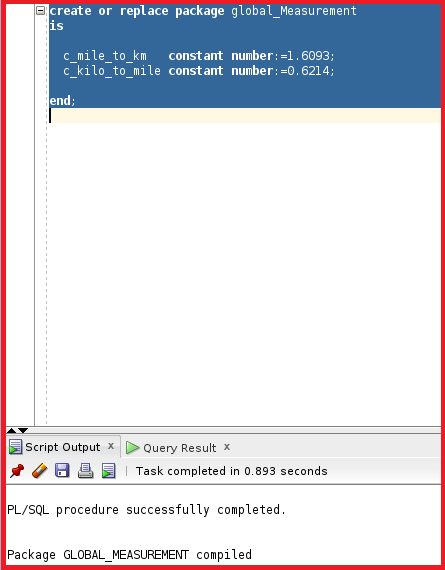So, the package is now successfully compiled. We can invoke the package by using execute.

execute dbms_output.put_line(’60 mile:=’||60* global_Measurement.c_mile_to_km||’ KM’ );

Let’s try to execute this statement and see the conversion.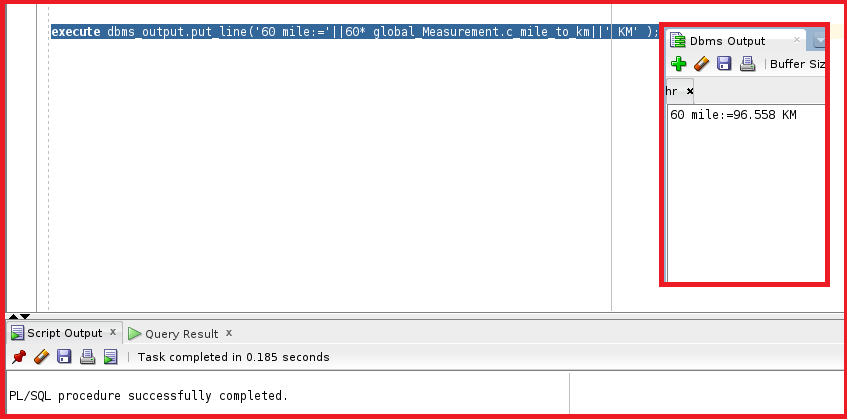We can see the 60 miles are converted to 96.558 Km. Let’s now check the other conversion.

execute dbms_output.put_line(‘100 KM:=’||100* global_Measurement.c_kilo_to_mile||’ Mile’ );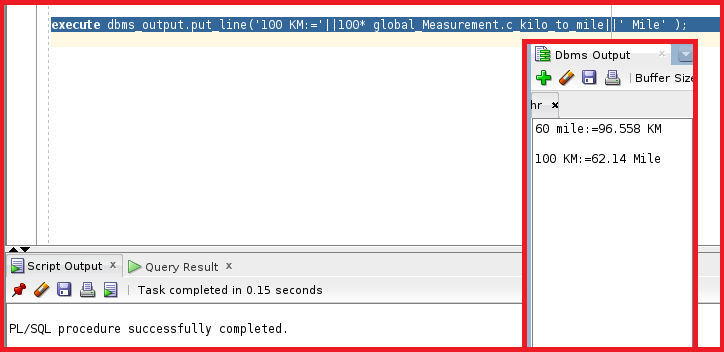So, this conversion is also working fine. We have learned that we can use packages without a package body in order to define global variables. Let’s do some other trick. Let’s create a function to read values from this package.

```create or replace function get_mile_to_km
( p_value number )
return number
is
begin
return p_value* global_Measurement.c_mile_to_km;
end;
```

We have created a function named get_mile_to_km which takes a parameter as input and multiple the value of the parameter with the constant value of the global_measurement package and c_mile_to_km constant value. This function will return the value of the constant number multiplied by the p_value. Let’s go ahead and compile this function.So, the function is compiled successfully. We can use this function in the select statement and try to get the kilometer conversion.

select get_mile_to_km(100) from dual;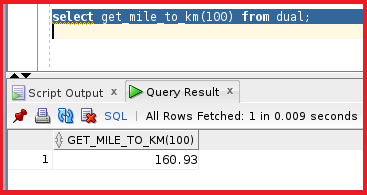We can see the output of 100 miles is converted to 160.93 km. The last step is can define a procedure or a function inside PL/SQL block, but this will be used only in this block.

```declare
function get_sysdate
return date
is
begin
return sysdate;
end;
begin
dbms_output.put_line(get_sysdate);
end;
```

This PL/SQL block will take sysdate as input and return the date as output. We have functions to describe the sysdate but we trying to show that we can declare the function inside the PL/SQL block. We can call this function as the local function and this is limited to only this block. Let’s go ahead and execute this block.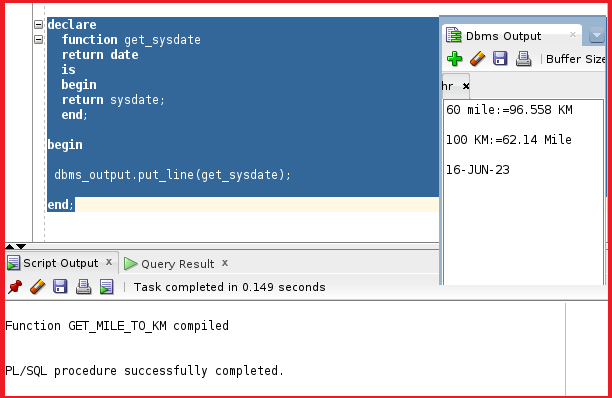So, this function is displaying the system date. So, we can create the package specification without the package body.

In the next article, I am going to discuss Package Components in Oracle with Examples. Here, in this article, I try to explain How to Create Package Specifications Without a Body in Oracle with Examples. I hope you enjoy this How to Create Package Specification without Body in Oracle article.